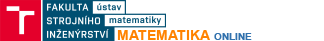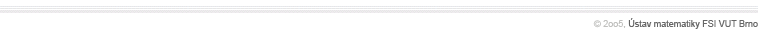Point sets and their topologyEuclidean space En, distance between points, Hölder's inequality, Minkowski's inequality, point neighbourhood, open sets, distance between sets, closed sets, border and interior of a set, finite and infinite unions and intersections of setsBasicsDefinition of an n-function, graph of an n-function, basic properties analogous to functions of one variable, composite functionsLimitsPoints of condensation, definition of the limit of an n-function, basic properties of limits of n-functions, equivalent definition using sequences of points, limits of two-functions, double limit, relationship between the double limit and limit of a two-function, continuous n-functionsPartial derivativesDefinition of a partial derivative, method of calculation, Schwartz theorem, partial derivatives of composite functionsDifferentialsTotal differential of an n-function, sufficient conditions for continuous functions, tangent plane and normal to the graph of a 2-function, total differential as a function, differentials of higher orders, using a total differential to calculate values of functionsImplicitly defined functionsDefinition of an implicitly defined function, when does an equation define implicitly a function?, higher-order partial derivatives of implicitly defined functionsLocal maxima/minimaDefinition of a local maximum and minimum of n-functions, necessary condition for a local minimum/maximum at a point, sufficient conditions for two-functions and three-functions to have local maxima/minimaRelative maxima/minimaConstraints, definition of relative maxima/minima, another sufficient condition for teh existence of local maxima/minima, method of Lagrange multipliers, finding relative maxima/minima for two- and three-functionsMaximum and minimum valuesRelationships between local maxima/minima and maximum/minimumvalues of an n-function, method of finding maximum/minimum values, examples